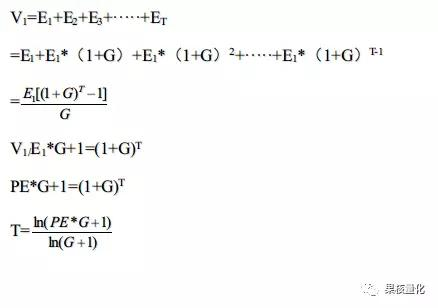PE的终极奥秘又是什么？

（当然以上你们可能都是知道的，觉得没什么卵用，下面看重点）T=ln100*0.3+1/ln1.3=13.09

T=ln50*0.2+1/ln1.2=13.15

T=ln20*0.15+1/ln1.15=9.92

T=PE=10

PE=10G=10%时，T=7.27

PE=20G=20%时，T=8.82

PE=30G=30%时，T=8.87

PE=40G=40%时，T=8.42

PE=50G=50%时，T=8.03

PE=100G=100%时，T=6.66